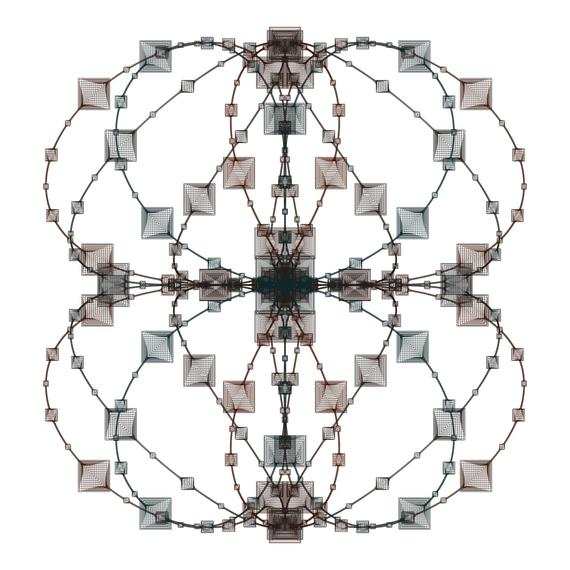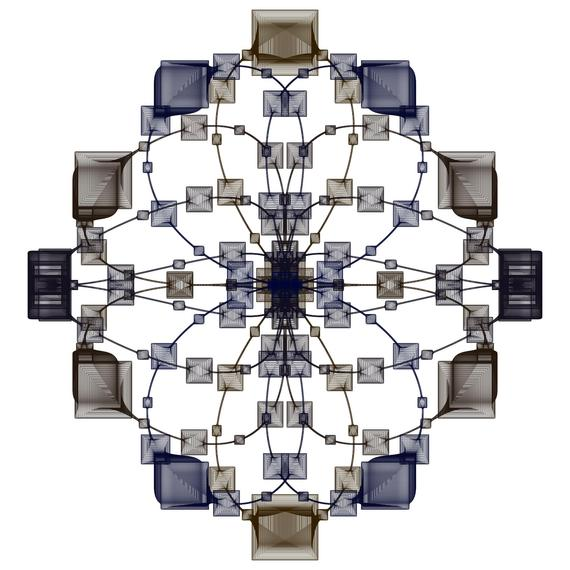# These Beautiful Images Are Created by Drawing Squares

In order to create beautiful images by drawing squares, it is very useful to use periodic functions. I have created the following images by drawing families of squares which are defined with trigonometric functions. At the end of this post you can see the mathematical descriptions of "16,000 Squares (3)" and "16,000 Squares (4)."

16,000 Squares (3)This image shows 16,000 squares. For each k=1, 2, 3, ... , 16000 the vertices of the k-th square are:

(X(k)-A(k), Y(k)-A(k)),
(X(k)+A(k), Y(k)-A(k)),
(X(k)+A(k), Y(k)+A(k)),
(X(k)-A(k), Y(k)+A(k)),

where

X(k)=sin(10πk/16000)(cos(18πk/16000))2,
Y(k)=(cos(14πk/16000))3,
A(k)=(1/400)+(1/25)(cos(64πk/16000)cos(192πk/16000))12+(1/70)(cos(192πk/16000)cos(384πk/16000))10+(1/120)(cos(384πk/16000)cos(1152πk/16000))10.

16,000 Squares (4)This image shows 16,000 squares. For each k=1, 2, 3, ... , 16000 the vertices of the k-th square are:

(X(k)-A(k), Y(k)-A(k)),
(X(k)+A(k), Y(k)-A(k)),
(X(k)+A(k), Y(k)+A(k)),
(X(k)-A(k), Y(k)+A(k)),

where

X(k)=sin(10πk/16000)(cos(14πk/16000))2,
Y(k)=cos(14πk/16000)(cos(10πk/16000))2,
A(k)=(1/400)+(1/12)(cos(14πk/16000)cos(42πk/16000))12+(1/30)(cos(96πk/16000)cos(192πk/16000))12+(1/60)(cos(192πk/16000)cos(384πk/16000))10.